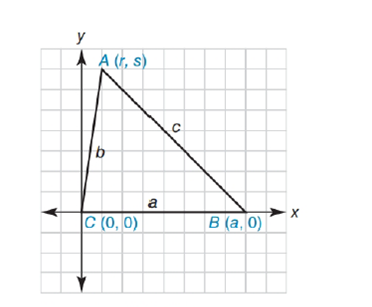Chapter 10.4, Problem 35EElementary Geometry For College St...

7th Edition
Alexander + 2 others
ISBN: 9781337614085

Solutions

Chapter
SectionElementary Geometry For College St...

7th Edition
Alexander + 2 others
ISBN: 9781337614085
Textbook Problem

Where point D lies on A B - , let C D - name the angle-bisector of ∠ A C B . In terms of a ,   b , and c , find A D and D B .Exercises 34. 35

To determine

To find:

AD and DB In terms of a, b, and c, where point D lies on AB-, let CD- name the angle-bisector of ACB.

Explanation

Given figure is,

The above figure shows the triangle ABC.

a is the length of CB

b is the length of AC

c is the length of AB

Angle Bisector theorem: It says that an angle bisector of a triangle will divide the opposite side into two segments that are proportional to the other sides of the triangle.

D is the point lies on AB and joining CD as shown in the figure.

Let BD is the length of x and AD is the length of c-x

BDBC=DAAC

xa=(c-x)b

Simplifying the above equation as below,

xab=c-x

bax=c-x

x=ab(c-x)

x=cab-xab

x+xab=cab

x1+ab=acb

xa+bb=a</

Still sussing out bartleby?

Check out a sample textbook solution.

See a sample solution

The Solution to Your Study Problems

Bartleby provides explanations to thousands of textbook problems written by our experts, many with advanced degrees!

Get Started

Find the minimum cost C (in dollars) given that 2(1.5C + 80) 2(2.5C 20)

Applied Calculus for the Managerial, Life, and Social Sciences: A Brief Approach

Simplify each power of i. i34

Trigonometry (MindTap Course List)

is the binomial series for:

Study Guide for Stewart's Multivariable Calculus, 8th

By definition the improper integral It is not an improper integral.

Study Guide for Stewart's Single Variable Calculus: Early Transcendentals, 8th

Find x. All dimensions are in inches

Mathematics For Machine Technology